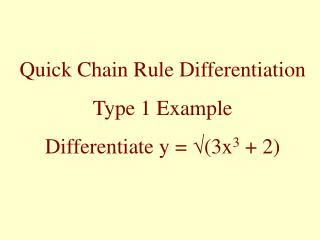DownloadDownload PresentationQuick Chain Rule Differentiation Type 1 Example Differentiate y = √ (3x 3 + 2)

# Quick Chain Rule Differentiation Type 1 Example Differentiate y = √ (3x 3 + 2)

Download Presentation## Quick Chain Rule Differentiation Type 1 Example Differentiate y = √ (3x 3 + 2)

- - - - - - - - - - - - - - - - - - - - - - - - - - - E N D - - - - - - - - - - - - - - - - - - - - - - - - - - -
##### Presentation Transcript

1. Quick Chain Rule Differentiation Type 1 Example Differentiate y = √(3x3 + 2)

2. First put it into indices y = √(3x3 + 2) = (3x3 + 2)½

3. y = √(3x3 + 2) = (3x3 + 2)½ Now Differentiate dy/dx = ½(3x3 + 2)-½9x2 Differentiate the inside of the bracket Differentiate the bracket, leaving the inside unchanged

4. A General Rule for Differentiating y = (f(x))n dy/dx = n(f(x))n-1 f´(x) Differentiate the bracket, leaving the inside unchanged Differentiate the inside of the bracket

5. Quick Chain Rule Differentiation Type 2 Example Differentiate y =e(x3+2)

6. y =e(x3+2) Differentiating dy/dx = 3x2 e(x3+2) Write down the exponential function again Multiply by the derrivative of the power

7. A General Rule for Differentiating dy/dx = f´(x) y =ef(x) ef(x) Multiply by the derrivative of the power Write down the exponential function again

8. Quick Chain Rule Differentiation Type 3 Example Differentiate y = In(x3 +2)

9. y = In(x3 +2) Now Differentiate dy/dx = 1 3x2 = 3x2 x3+ 2 x3+ 2 One over the bracket Times the derrivative of the bracket

10. A General Rule for Differentiating y = In(f(x)) dy/dx = 1 f´(x) = f´(x) f(x)f(x) Times the derrivative of the bracket One over the bracket

11. y dy/dx (f(x))n n(f(x))n-1 f´(x) e(f(x)) f´(x)e(f(x)) In(f(x)) f´(x) f(x) Summary